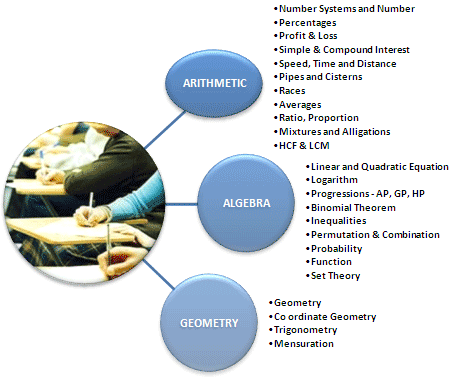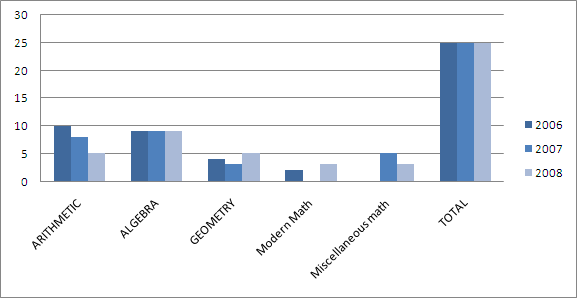# QUANTITATIVE ABILITYCat Exam
Explore more-CAT
KI Unique
Test Yourself
Help

## QUANTITATIVE ABILITY

The Quantitative Ability Section tests the ability of problem solving in a candidate. This section covers various topics from Mathematics and Data sufficiency in general. These topics are as under:• The Basic mathematics from 9th to 12th should be clear.
• As the basic formulae and types of questions related to these topics should be clear before appearing for the CAT exam.
• More often than not, students who take CAT find the quant section as the toughest one.
• Number system further consists of divisibility property, Integers, even and odd numbers etc.

### ARITHMETIC

• About 15-20% of total questions fall under this category.
• However Number Theory along with algebra formulates the major chunk of questions. Range could be 55%- 60%.
• Set Theory and problems related to Time, Distance and Speed constitute major percentage of questions in this section.
• This section may also contain some miscellaneous problems but they will be not more than one or two and will be just to check out mathematical aptitude of the candidate. One can’t learn to solve such problems.
• It is better to avoid miscellaneous problems, as negative marking can cause problems along with time wastage in this case.

### ALGEBRA

• As already mentioned, algebra along with number theory constitutes the major chunk of questions in this section of CAT.
• Topics that you need to look at are Permutations and Combinations, Probability (very basic, including die and card problems and perhaps Bayes' theorem), Functions, Progressions (A.P, G.P. H.P. and A.G.P), Logarithms, and Equations (Quadratic and Linear/Simultaneous).

### GEOMETRY

• Maximum weightage is given to geometry, although every CAT paper will have 3-4 questions on mensuration, as well as a couple of questions on coordinate geometry, totaling about 25-30% of questions in the QA section.
• Topics that need to be covered in geometry are basic theorems involving triangles, circles and parallel lines.
• A common type of question that is often asked in CAT is to find the value of certain angles or length of certain sides. Therefore, make sure that you cover topics such as congruency and similarity of triangles.
• The only things that you need to do in coordinate geometry are straight lines and circles. Don't go into conic sections and other advanced topics.
• Mensuration problems are calculation intensive, and require lots of practice.### DATA SUFFICIENCY

• Data Sufficiency problems can come from any of the three heads, and are in the form of a question followed by two statements.
• You need to answer whether you can solve the problem using the statements individually, or using both, or whether you cannot solve the problem using the information provided.
• These problems are generally tricky.
• Candidate will be presented with a statement that is followed by two other statements; the candidate has to tell whether the given statements are enough to solve the question.
• This type of question tests confirms candidate’s ability to understand and ability to identify the missing data of problem.
Thus Quantitative area needs proper PRACTICE along with clever and smart hard work.
Copy Right © 2012 by Knowledge Icon
Website Designed & Developed By Dream Business Systems